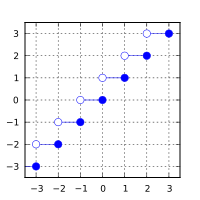/C++

# std::ceil, std::ceilf, std::ceill

Defined in header `<cmath>`
(1)
```float       ceil ( float num );
double      ceil ( double num );
long double ceil ( long double num );```
(until C++23)
```constexpr /* floating-point-type */
ceil ( /* floating-point-type */ num );```
(since C++23)
`float       ceilf( float num );`
(2) (since C++11)
(constexpr since C++23)
`long double ceill( long double num );`
(3) (since C++11)
(constexpr since C++23)
Defined in header `<cmath>`
```template< class Integer >
double      ceil ( Integer num );```
(A) (constexpr since C++23)
1-3) Computes the least integer value not less than `num`. The library provides overloads of `std::ceil` for all cv-unqualified floating-point types as the type of the parameter. (since C++23)
 `double` (since C++11)

### Parameters

 num - floating point or integer value

### Return value

If no errors occur, the smallest integer value not less than `num`, that is ⌈num⌉, is returned.

Return value`num`

### Error handling

Errors are reported as specified in `math_errhandling`.

If the implementation supports IEEE floating-point arithmetic (IEC 60559),

• The current rounding mode has no effect.
• If `num` is ±∞, it is returned unmodified
• If `num` is ±0, it is returned, unmodified
• If `num` is NaN, NaN is returned

`FE_INEXACT` may be (but is not required to be) raised when rounding a non-integer finite value.

The largest representable floating-point values are exact integers in all standard floating-point formats, so this function never overflows on its own; however the result may overflow any integer type (including `std::intmax_t`), when stored in an integer variable. It is for this reason that the return type is floating-point not integral.

This function (for double argument) behaves as if (except for the freedom to not raise `FE_INEXACT`) implemented by the following code:

```#include <cfenv>
#include <cmath>

#pragma STDC FENV_ACCESS ON

double ceil(double x)
{
int save_round = std::fegetround();
std::fesetround(FE_UPWARD);
double result = std::rint(x); // or std::nearbyint
std::fesetround(save_round);
return result;
}```

The additional overloads are not required to be provided exactly as (A). They only need to be sufficient to ensure that for their argument `num` of integer type, `std::ceil(num)` has the same effect as `std::ceil(static_cast<double>(num))`.

### Example

```#include <cmath>
#include <iostream>

int main()
{
std::cout << std::fixed
<< "ceil(+2.4) = " << std::ceil(+2.4) << '\n'
<< "ceil(-2.4) = " << std::ceil(-2.4) << '\n'
<< "ceil(-0.0) = " << std::ceil(-0.0) << '\n'
<< "ceil(-Inf) = " << std::ceil(-INFINITY) << '\n';
}```

Output:

```ceil(+2.4) = 3.000000
ceil(-2.4) = -2.000000
ceil(-0.0) = -0.000000
ceil(-Inf) = -inf```

 floorfloorffloorl (C++11)(C++11) nearest integer not greater than the given value (function) trunctruncftruncl (C++11)(C++11)(C++11) nearest integer not greater in magnitude than the given value (function) roundroundfroundllroundlroundflroundlllroundllroundfllroundl (C++11)(C++11)(C++11)(C++11)(C++11)(C++11)(C++11)(C++11)(C++11) nearest integer, rounding away from zero in halfway cases (function) nearbyintnearbyintfnearbyintl (C++11)(C++11)(C++11) nearest integer using current rounding mode (function) rintrintfrintllrintlrintflrintlllrintllrintfllrintl (C++11)(C++11)(C++11)(C++11)(C++11)(C++11)(C++11)(C++11)(C++11) nearest integer using current rounding mode with exception if the result differs (function) C documentation for `ceil`